物理层

通信基础

信道、信号、带宽、码元、波特速率、信源与信宿等基本概念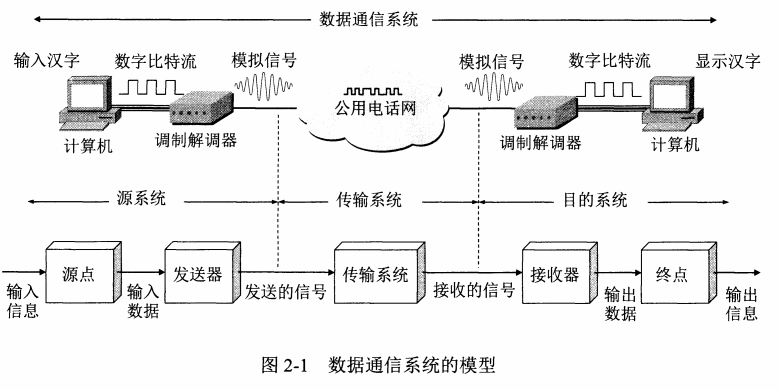• 信道：信道是信号的传输媒介，是一条线路的逻辑部件。一条通信电路玩玩包含一条发生信道和一条接收信道。
1. 单向通信（单工通信
2. 双向交替通信（半双工通信
3. 双向同时通信（全双工通信
• 数据：信息的承载实体。
• 信号：数据的电磁或电气表现。
• 带宽：媒介中信号可使用的最高频率和最低频率之差，或者说是频带的宽度;另一个定义是信道中数据的传送速率。
• 码元：在使用时间域(简称时域)的波形表示数字信号时，代表不同离散数值的基本波形。
• 波特：单位时间内传输的码元数。
• 比特率：单位时间内传输的比特数。
• 信息传播过程简单地描述为：信源→信道→信宿。

奈奎斯特定理与香农定理

• 奈奎斯特(Nyquist)无噪声下的码元速率极限值 $B$ 与信道带宽 $H$ 的关系：

最大数据传输率 $B=2H\ (Baud)$

$Band$ 波特：1波特表示数字通信系统每秒传输一个码原，码原可以是多进制的、可以表示多个比特的数据

奈奎斯特公式–无噪信道传输能力公式：$C=2H\ log₂N \ (bps)$

式中 $H$ 为信道的带宽，即信道传输上、下限频率的差值，单位为 $Hz$ ; $N$ 为一个码元所取的离散值个数。

• 香农公式–带噪信道容量公式：

$C=H\ log₂(1+S/N)\ (bps)$

式中 $S$ 为信号功率，$N$ 为噪声功率，$S/N$ 为信噪比，通常把信噪比表示成 $10lg(S/N)$ 分贝 $(dB)$。

给出的信噪比通常是多少分贝，需要注意 $S/N=10^{分贝/10}$ 的换算

编码与调制

• 数字数据编码为数字信号

1. 不归零制：用一个相位表示 $1\ Bit$ 数据，正电平代表1,负电平代表0。
2. 归零制：用一个相位表示 $1\ Bit$ 数据，正脉冲代表1,负脉冲代表0。
3. 曼期特编码：用两个相位表示 $1\ Bit$ 数据，一相位高一相位低表示 $1$，反之表示 $0$
4. 查分曼期特编码：用两个相位表示 $1\ Bit$ 数据，改变为 $1$，不变为 $0$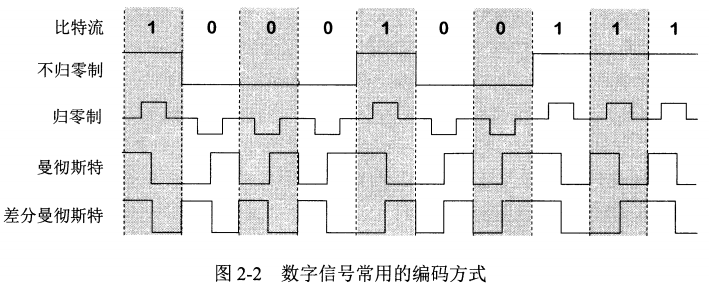• 数字数据调制为模拟信号

1. 调幅(AM)
2. 调频(FM)
3. 调相(PM)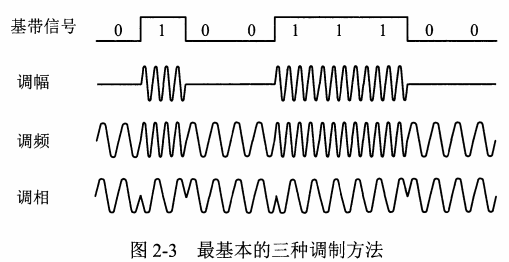电路交换、报文交换与分组交换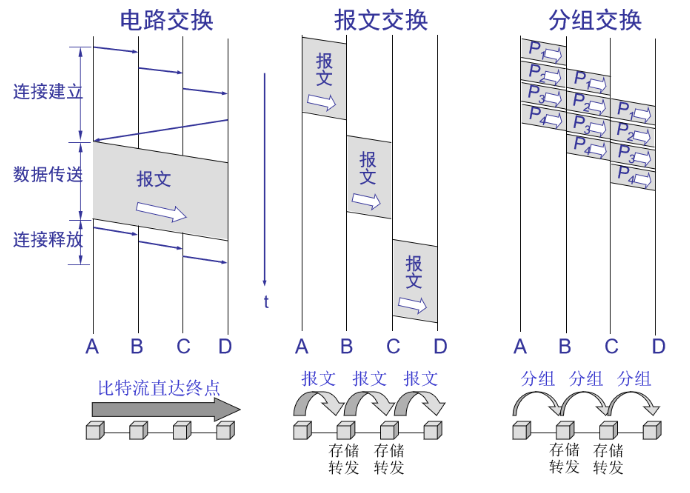• 电路交换：在通信之前要在通信双方之间建立一条被双方独占的物理通路( 由通信双方之间的交换设备和链路逐段连接而成)
• 报文交换：以报文为数据交换的单位，报文携带有目的地址、源地址等信息，在交换结点采用存储转发的传输方式。
• 分组交换：仍采用存储转发方式，它将一个大的数据块分割成若千个小数据块，再加上- - 些必要的控制信息(如源地址、目的地址等)，构成分组。网络结点根据控制信息把分组送到下一结点，下一结点接收到分组后，暂时保存下来并排队等待传输，然后根据分组控制信息选择它的下一个结点，直到到达目的结点。

传输介质

双绞线、同轴电缆、光纤与无线传输介质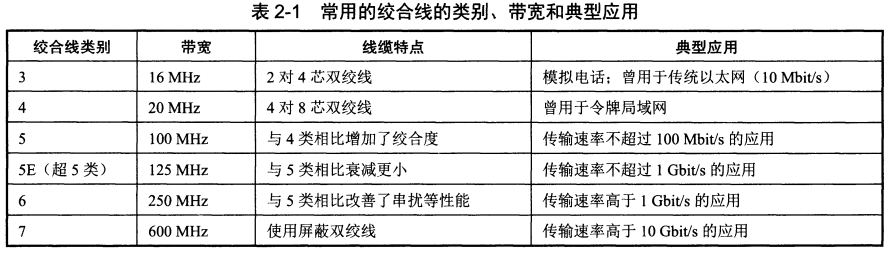物理层接口的特性

• 机械特性：定义物理连接的边界点，即接插装置。规定物理连接时所采用的规格、引线的数目、引脚数目和排列情况等。
• 电气特性：线路上信号的电压高低、阻抗匹配、传输速率和距离限制等。
• 功能特性：指明某条线上出现的某-电平的电压表示何种意义。
• 过程特性：指名对于不同功能的各种可能事件的出现顺序。